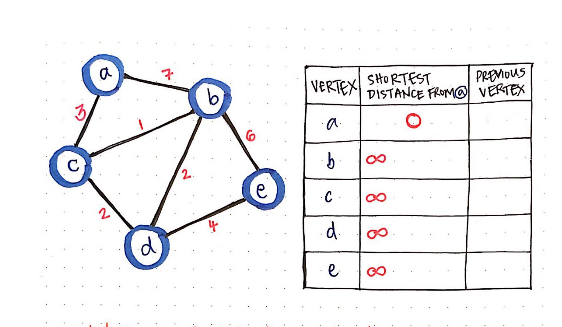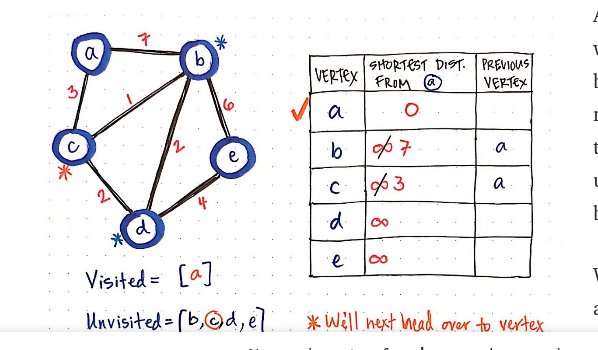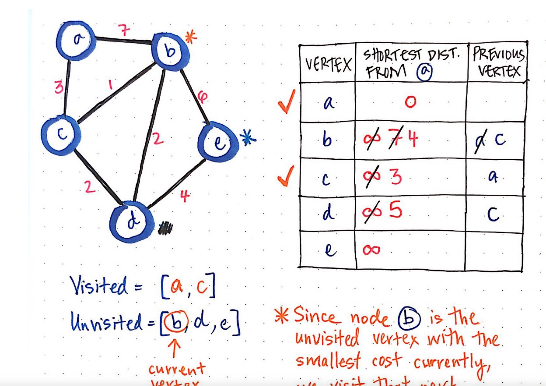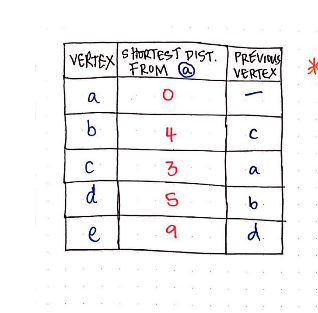### This algorithm finds shortest path from a graph structures which has 2 elements known as,

1. Nodes/Vertices
2. Edges

### Edge:

A node (or vertex) is a discrete position in the graph.And an edge (or connection) is a link between two vertices that can be either directed or undirected and may have a cost associated with it.

The 2 vertices connected by edge are source and destination.The length of edge denotes distance/cost between 2 nodes.

##### Code Implementation:

class Edge

attr_accessor :src, :dst, :length

def initialize(src, dst, length = 1)

@src = src

@dst = dst

@length = length

end

end

##### Graph:

Graph is an array of such edges.It may contain loops.

It can be initialized as,

def initialize

@edges = []

end

#### Connecting 2 vertices by creating a edge between them with certain length,

def connect(src, dst, length = 1)

unless self.include?(src)

raise ArgumentException, “No such vertex: #{src}”

end

unless self.include?(dst)

raise ArgumentException, “No such vertex: #{dst}”

end

@edges.push Edge.new(src, dst, length)

end

#### Rules of Dijkstra’s game

1. From the starting node, visit the vertex with the smallest known distance.
2. Once we’ve moved to the smallest-cost vertex,check each of its neighbouring nodes.
3. Calculate the distance/cost from the neighbouring nodes by summing the cost of edges leading from the start vertex.
4. If the distance to a vertex we are checking is less than a known distance, update the shortest distance for that vertex.

#### Consider below ex:

Step 1:Initialize the distance as infinite from start node.Visited =[] unvisited=[a,b,c,d,e]

###The distance to node b is currently marked as 7 . But, there is a shorter path to b, which goes through c, and has a cost of only 4. So, the table will be updated with shorter paths.#### After visiting all nodes, the table becomesSo we have visited all nodes. The shortest path for all nodes from any node can be found from above table.

Code implementation:

def dijkstra(src, dst = nil)

distances = {}

previouses = {}

self.each do |vertex|

distances[vertex] = nil # Infinity

previouses[vertex] = nil

end

distances[src] = 0

vertices = self.clone

until vertices.empty?

nearest_vertex = vertices.inject do |a, b|

next b unless distances[a]

next a unless distances[b]

next a if distances[a] < distances[b]

b

end

break unless distances[nearest_vertex] # Infinity

if dst and nearest_vertex == dst

return distances[dst]

end

neighbors = vertices.neighbors(nearest_vertex)

neighbors.each do |vertex|

alt = distances[nearest_vertex] + vertices.length_between(nearest_vertex, vertex)

if distances[vertex].nil? or alt < distances[vertex]

distances[vertex] = alt

previouses[vertices] = nearest_vertex

# decrease-key v in Q # ???

end

end

vertices.delete nearest_vertex

end

if dst

return nil

else

return distances

end

end

#Creating graph

graph = Graph.new

(1..6).each {|node| graph.push node }

#Connecting 2 nodes

graph.connect 1, 2, 7

#connect all nodes like above

#Find cost/distance

graph.length_between(2, 1)

#Find shortest path

graph.dijkstra(1, 5)